Editor Ratings:
User Ratings:
[Total: 0 Average: 0]

This article covers 5 free Magic Square Generator websites. These websites let you generate the magic square of various grid size.

In a magic square, the sum of each row, each column, and at least two diagonals is always equal. This sum is known as the magic sum.

Enter the magic sum and these websites will generate magic square for that sum. Each Magic Square Generator has something different than others. Let’s discuss them one by one and see what they have to offer.

## MindMagician.org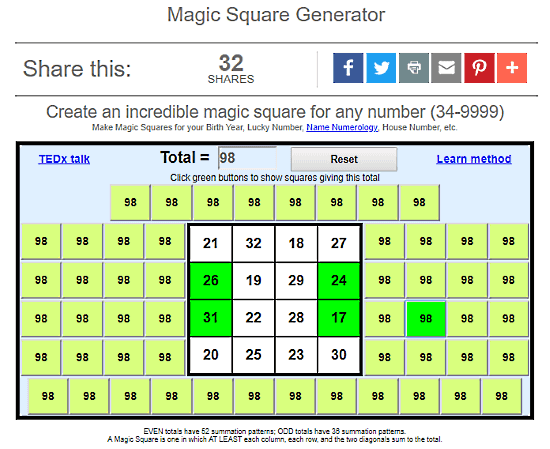MindMagician.org is created by a popular magician, psychologist, and author Michael Daniels. This website has a magic square generator where you can generate a 4×4 magic square for a magic sum value from 34 to 9999. Michael Daniels also gave a TEDx talk on this magic square generator. The unique thing about this magic square generator is that it generates magic square with summation patterns i.e. the sum of any four cells is same as the magic sum. Magic square with even magic sum has 52 summation patterns and in case of an odd magic sum, it has 36 summation patterns.

Try Mindmagician’s Magic Square Generator here.

## Dcode.fr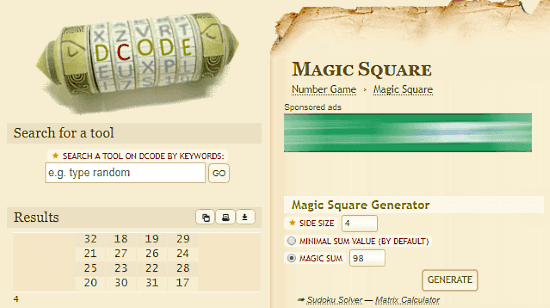Dcode.fr offers various tools for games, puzzles, text encryptions, etc. Its Magic Square Generator tool lets you generate magic square of up to 25 grid size. After mentioning grid size, you can generate magic square for a specific magic sum value or for a minimal magic sum value. If you only mention the grid size, it creates the magic square with minimal sum value by default.

Unlike others, this tool also uses negative and decimal values to generate magic square which gives you the flexibility to generate magic square for any value of the magic sum.

Give Dcode’s Magic Square Generator a try here.

## MarkFarrar.co.uk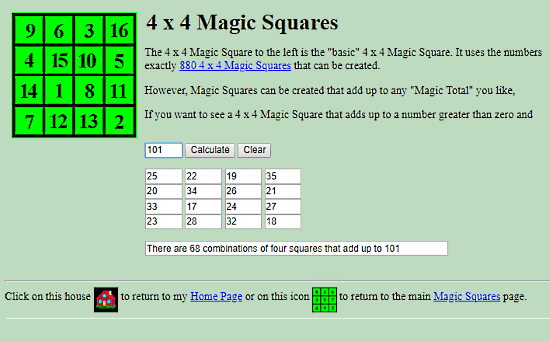MarkFarrar.co.uk has an online Magic Square Generator where you can generate 4×4 magic squares. Here, you can generate magic square for any magic sum value in between 0 to 1000. Just enter the magic sum and click ‘calculate‘ and it will instantly generate a magic square with that magic sum value. It also tells you how many 4 cells combinations are there which add up to the sum value.

You can try MarkFarrar’s Magic Square Generator here.

## Grogono.com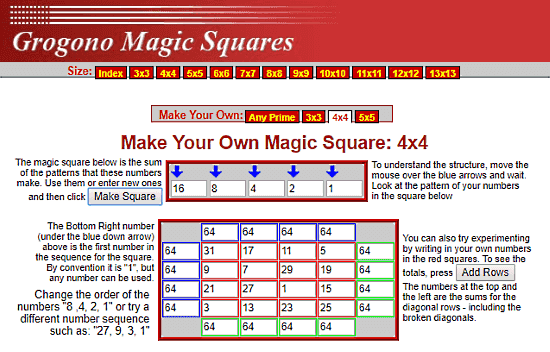Grogono.com generates magic squares of any grid size between 3 to 97. The magic squares of 3, 4 and 5 grid size are the main focus here. For these grid sizes, you can generate magic square by defining a pattern rather than using magic sum value as input. Each cell value in the magic square is either a pattern value or the sum of two or more pattern values.

You can also experiment by entering your own numbers in the square and it will calculate the sum for the square. Depending on your numbers, it would or would not be a magic square.

Give Grogono‘s Magic Square Generator a try here.

## MindYourDecisions.com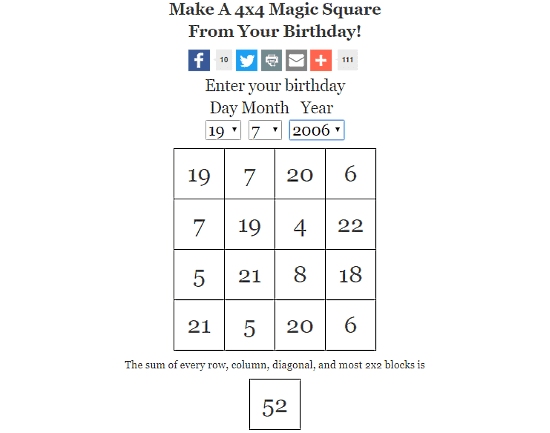MindYourDecisions.com has an online Magic Square Generator that generates a 4×4 magic square based on your birth date. Give it your birth date or any date (day, month & year) as input and it will generate a 4×4 magic square for you. It also shows you the magic sum of the square. To make this possible, it uses both positive as well as negative integer values in the square.

Try MindYourDecisions’s Magic Square Generator here.

### Closing Words:

All these Magic Square Generator websites are pretty simple to use. You can easily generate magic square using any of them.

 Editor Ratings: User Ratings:[Total: 0 Average: 0] Free/Paid: Free